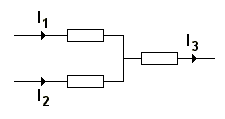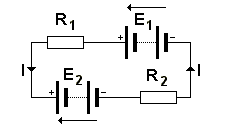# Kirchoff's Laws

Kirchoff's voltage and current laws basically state that voltage or current in a circuit must be accounted for - it cannot just 'disappear'. The laws are as follows:

#### Kirchoff's Current Law:

Kirchoff's Current Law states that 'the algebraic sum of the current meeting at any point in a circuit is zero'.

For example:I3 = I1 + I2

or I1 + I2 - I3 = 0 (currents towards point designated as positive, those away from point negative).

In other words the sum of all currents entering a junction must equal the sum of those leaving it.

#### Kirchoff's Voltage Law:

Kirchoff's Voltage Law states that 'in travelling round any closed mesh (section) of a network (circuit) , the algebraic sum of the emfs (voltages) acting in the mesh is equal to the algebraic sum of the IR voltage drops for the individual resistance in the mesh.'

For example:Working anticlockwise:

IR1 + IR2 = E1 - E2

Working clockwise:

-IR1 - IR2 = E2 - E1

In other words the sum of all voltage sources must equal the sum of all voltages dropped across resistances in the circuit, or part of circuit.